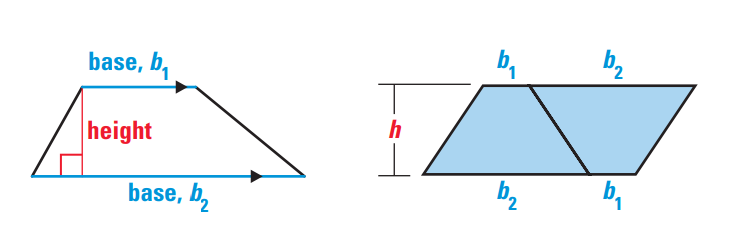# Trapezoid Area Calculator

Trapezoid area calculator is an online tool to calculate area of a trapezoid, when the two base b1, b2 and height h is known. A trapezoid is a quadrilateral with exactly one pair of parallel sides. The parallel sides are called bases of the trapezoid. The nonparallel sides are referred to as the legs of the trapezoid.

## Definition - What is Trapezoid?

The definition that most north American mathematicians use for trapezoid is a four-sided shape with exactly one pair of opposite sides parallel.

A trapezoid is a quadrilateral that has one pair of sides which are parallel. These two sides are called the bases of the trapezoid. The height of a trapezoid is a segment that connects the one base of the trapezoid and the other base of the trapezoid and is perpendicular to both of the bases.

### Trapezoid Area Formula

Recall that the parallel sides of a trapezoid are called the bases of the trapezoid, with lengths denoted by b1 and b2. The shortest distance between the bases is the height of the trapezoid.Suppose that two congruent trapezoids with bases b1 and b2 and height h are arranged to form a parallelogram. The area of the parallelogram is h x (b1 + b2). Because the two trapezoids are congruent, the area of one of the trapezoids is half the area of the parallelogram.b1=the one base of the trapezoid

b2=the other base of the trapezoid

h=the height of the trapezoid

Altitude

An altitude of a trapezoid is a segment drawn from any point on one of the parallel sides (base) perpendicular to the opposite side (the other base). An infinite number of altitudes may be drawn in a trapezoid.

Median

A median of a trapezoid is the segment that joins the midpoints of the nonparallel sides (legs).

## Latest Blog topics### Spreading Knowledge Across the World

USA - United States of America  Canada  United Kingdom  Australia  New Zealand  South America  Brazil  Portugal  Netherland  South Africa  Ethiopia  Zambia  Singapore  Malaysia  India  China  UAE - Saudi Arabia  Qatar  Oman  Kuwait  Bahrain  Dubai  Israil  England  Scotland  Norway  Ireland  Denmark  France  Spain  Poland  and  many more....